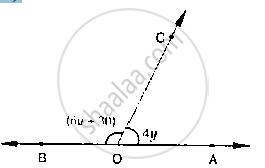# What Value of Y Would Make Aob a Line in Below Fig, If ∠Aoc = 4y and ∠Boc = (6y + 30) - Mathematics

What value of y would make AOB a line in below fig, if ∠AOC = 4y and ∠BOC = (6y +
30)#### Solution

Since ∠AOC, ∠BOC are linear pair

⇒ ∠AOC + ∠BOC = 180°

⇒ 6 y + 30 + 4 y = 180°

10 y + 30 = 180°

⇒ 10 y = 180° - 30°

10 y = 150°

⇒ y = (150°)/10

⇒ y = 15°

Concept: Pairs of Angles
Is there an error in this question or solution?
Chapter 10: Lines and Angles - Exercise 10.2 [Page 15]

#### APPEARS IN

RD Sharma Mathematics for Class 9
Chapter 10 Lines and Angles
Exercise 10.2 | Q 13 | Page 15

Share